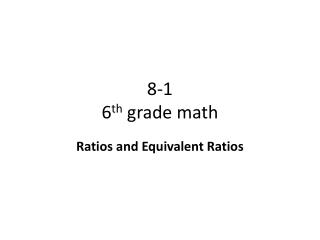DownloadDownload Presentation# 8-1 6 th grade math

Télécharger la présentation## 8-1 6 th grade math

- - - - - - - - - - - - - - - - - - - - - - - - - - - E N D - - - - - - - - - - - - - - - - - - - - - - - - - - -
##### Presentation Transcript

1. 8-16th grade math Ratios and Equivalent Ratios

2. Objective • To read and write ratios and equivalent ratios • Why? To think of ratios as comparisons of two quantities.

3. California State Standards NS 1.2 : Interpret and use ratios in different contexts (e.g., batting averages, miles per hour) to show the relative sizes of two quantities, using appropriate notations (x/y, x to y, x:y) MR 1.1: Analyze problems by identifying relationships …

4. Vocabulary • Ratio • A comparison of two quantities • 12 to 9, 12/9, 12:9 • Equivalent ratio • When both terms are multiplied or divided by a number other than zero. Ratios that represent the same rate or make the same comparison. • 12/9 = 108/81 (x by 9)

5. How to Compare Ratios 1) Cross multiply the denominator of the first to the numerator of the second. Write the number on top. (IF ratio is written in non-fraction form, re-write into fraction form. Order MATTERS!) 2) Cross multiply the denominator of the second to the numerator of the first. Write the number on top. 3) Compare the two numbers. 149 • 6 84 108 14 ↖ ↗ 9 12 ↗↖ 6 84 < 108 14 < 9 • 6

6. Another Way to Compare Ratios • Make equivalent fractions. • Compare numerators. • Check for reasonableness. 149 • 6 1418 • 12 14 < 18 14 < 9 • 6

7. To Find the Missing Equivalent Ratio Number • Cross multiply the numerator and denominator of the two known numbers. • Divide by the last number. 3. Check your work. Does the answer seem reasonable. 4 = 12 9 X 9 x 12 = 108 27 4 = 12 9 27

8. To Find the Missing Equivalent Ratio Number • Look at the known numerator and denominator. Decide what number to multiply or divide by to make the equivalence. • If ‘moving’ in the same direction, use the same operation as in Step 1. • If ‘moving’ in the opposite direction, use the opposite operation in Step 1. 3. Check your work. Does the answer seem reasonable. 4 = 12 • X 4 → 12 (by x 3) 9 → x 3 = 27 4 = 12 9 27 ----------------------------------------------- 4 = 12 X 27 4 → 12 (by x 3) 27 ← ÷ 3 = 9 4 = 12 9 27

9. Try It! • 5 : 8 ___ 15 : 24 • 3 ____ 9 12 36 3) 4 ____ 16 6 20 4) 9 to 8 ____ 45 to 40 • 5 = 15 8 24 2) 3 = 9 12 36 3) 4 ≠ 16 6 20 4) 9 = 45 8 40

10. Objective Review • To read and write ratios and equivalent ratios • Why? You can now think of ratios as comparisons of two quantities. • A ratio is a comparison of two quantities. Order is important in writing a ratio.

11. Independent Practice • Complete problems 6-14 • Copy original problem first. • Show all work! • If time, complete Mixed Review: 15-19 • If still more time, work on Accelerated Math.